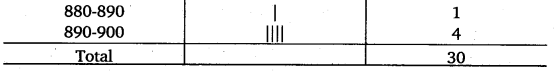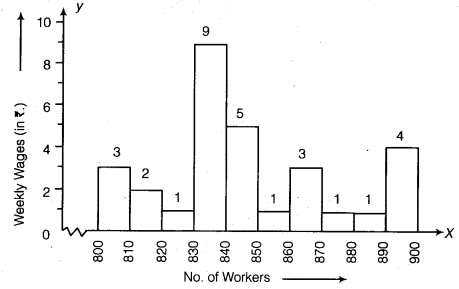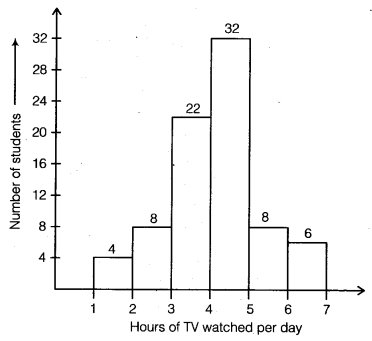# Class 8 Maths NCERT Solutions for Chapter – 5 Data Handling Ex – 5.1

## Data Handling

Question 1.
For which of these would you use a histogram to show the data?
(a) The number of letters for different areas in a postman’s bag.
(b) The height of competitors in an athletics meet.
(c) The number of cassettes produced by 5 companies.
(d) The number of passengers boarding trains from 7:00 a.m. to 7:00 pan. at a station.
Give reasons for each.

Solution:
In (b) and (d), data can be divided into class intervals. So, their histograms can be drawn.

Question 2.
The shoppers who come to a departmental store are marked as: man (M), woman (W), boy (B) or Girl (G). The following list gives the shoppers who came during the first hour in the morning. W W W G B W W M G G M M W W W W G B M W B G G M W W M M W W W M W B W G M W W W W G W M M W W M W G W M G W M M B G G W
Make a frequency distribution table using tally marks. Draw a bar graph to illustrate it.

Solution:
We arrange the data in a table using tally marks as :Question 3.
The weekly wages (in ?) of 30 workers in a factory are :
830, 835, 890, 810, 835, 836, 869, 845, 898, 890, 820, 860, 832, 833, 855, 845, 804, 808, 812, 840, 885, 835, 835, 836, 878, 840, 868, 890, 806,840.
Using tally marks make a frequency table with intervals as 800-810, 810-820 and so on.

Solution:
Let us make a grouped frequency table as under :Question 4.
Draw a histogram for the frequency table made for the data in question 3, and answer the following questions.
(i)
Which group has the maximum number of workers?
(ii) How many workers earn? 850 and more?
(iii) How many workers earn less than? 850?

Solution:
The histogram to the data of question 3 is as :From the above histogram, clearly,
(i) The group 830-840 has the maximum number of workers.
(ii) The number of workers who earn ₹ 850 and more are 1 + 3 + 1 + 1 + 4, i.e., 10.
(iii) The number of workers who earn less than ₹ 850 are 3 + 2 + 1 + 9 + 5, i.e., 20.

Question 5.The number of hours for which students of a particular class watched television during holidays is shown through the given graph (on previous page).

(i) For how many hours did the maximum number of students watch TV?
(ii) How many students watched TV for less than 4 hours?
(iii) How many students spend more than 5 hours in watching TV?

Solution:
Clearly from the given histogram, we find that
(i) The maximum number of students watch TV for 4 – 5 hours.
(ii) The number of students who watch TV for less than 4 hours are 4 + 8 + 22 = 34.
(iii) The number of students who spend more than 5 hours in watching TV are 8 + 6 = 14.